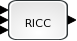Scilab Home page | Wiki | Bug tracker | Forge | Mailing list archives | ATOMS | File exchange
Change language to: English - Français - Português -

Please note that the recommended version of Scilab is 6.0.2. This page might be outdated.
However, this page did not exist in the previous stable version.

# RICC

Riccati Equation

### Block Screenshot### Description

This block computes the solution of Riccati equation using different method and for both case continuous and discrete.

The Riccati equation in continuous time is:

A*X+X*A+C-X*D*X=0

The Riccati equation in discrete time is:

A*X*(inv(In-D))*A-X+C=0

where A is an NxN matrix, it is the first input of the block, C and D are two NxN symmetrics matrices and are respectively the second and third input of the RICC block. X represent the output of the block, it is also a NxN matrix.

The user can choose between two methods of computation. For the continuous time he can use even the Schur method or the matrix sign function approach method, by setting the Model parameter to 1 or 2. For the discrete time, the models are the Schur method and the inverse free spectral decomposition method.

### Dialog box• Type (1=Cont 2=Disc)

For continuous time signal set this parameter to 1. For discrete input time set it to 2.

Properties : Type 'vec' of size 1.

• Model(1=Schr 2=sign(cont) inv(disc))

To use the Shur method in computation set this parameter to 1. To use matrix sign function approach in continuous case or the inverse free spectral decomposition method in discrete case set this parameter to 2.

Properties : Type 'vec' of size 1.

### Default properties

• always active: no

• direct-feedthrough: yes

• zero-crossing: no

• mode: no

• regular inputs:

- port 1 : size [-1,-1] / type 1

- port 2 : size [-1,-1] / type 1

- port 3 : size [-1,-1] / type 1

• regular outputs:

- port 1 : size [-1,-1] / type 1

• number/sizes of activation inputs: 0

• number/sizes of activation outputs: 0

• continuous-time state: no

• discrete-time state: no

• object discrete-time state: no

• name of computational function: ricc_m

### Interfacing function

• SCI/modules/scicos_blocks/macros/MatrixOp/RICC.sci

### Computational function

• SCI/modules/scicos_blocks/src/c/ricc_m.c

### Authors

Fady NASSIF - INRIA